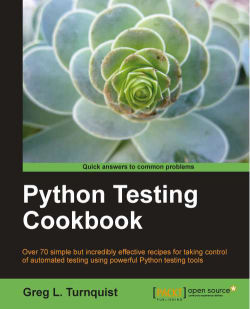### Python Testing Cookbook

By Greg L. Turnquist

• Constantly updated with 100+ new titles each month
• Breadth and depth in over 1,000+ technologies

Are you looking at new ways to write better, more efficient tests? Are you struggling to add automated testing to your existing system? The Python unit testing framework, originally referred to as "PyUnit" and now known as unittest, is a framework that makes it easier for you to write automated test suites efficiently in Python. This book will show you exactly how to squeeze every ounce of value out of automated testing.

The Python Testing Cookbook will empower you to write tests using lots of Python test tools, code samples, screenshots, and detailed explanations. By learning how and when to write tests at every level, you can vastly improve the quality of your code and your personal skill set. Packed with lots of test examples, this will become your go-to book for writing good tests.

This practical cookbook covers lots of test styles including unit-level, test discovery, doctest, BDD, acceptance, smoke, and load testing. It will guide you to use popular Python tools effectively and discover how to write custom extensions. You will learn how to use popular continuous integration systems like Jenkins (formerly known as Hudson) and TeamCity to automatically test your code upon check in. This book explores Python's built-in ability to run code found embedded in doc strings and also plugging in to popular web testing tools like Selenium. By the end of this book, you will be proficient in many test tactics and be ready to apply them to new applications as well as legacy ones.

Publication date:
May 2011
Publisher
Packt
Pages
364
ISBN
9781849514668

## Chapter 1. Using Unittest To Develop Basic Tests

In this chapter, we will cover:

• Asserting the basics

• Setting up and tearing down a test harness

• Running test cases from the command line

• Running a subset of test case methods

• Chaining together a suite of tests

• Defining test suites inside the test case

• Retooling old test code to run inside unittest

• Breaking down obscure tests into simple ones

• Testing the edges

• Testing corner cases by iteration

## Introduction

Testing has always been a part of software development. However, the world was introduced to a new concept called automated testing when Kent Beck and Erich Gamma introduced JUnit for Java development (http://junit.org). It was based on Kent's earlier work with Smalltalk and automated testing (http://www.xprogramming.com/testfram.htm). In this day and age, automated testing has become a well-accepted concept in the software industry.

A Python version, originally dubbed PyUnit, was created in 1999 and added to Python's standard set of libraries later in 2001 in Python 2.1 (http://docs.python.org/library/unittest.html). Since then, the Python community referred to it as unittest, the name of the library imported into the test code.

Unittest is the foundation of automated testing in the Python world. In this chapter, we will explore the basics of testing and asserting code functionality, building suites of tests, test situations to avoid, and finally testing edges, and corner cases.

For all the recipes in this chapter, we will use `virtualenv` (http://pypi.python.org/pypi/virtualenv) to create a controlled Python runtime environment. Unittest is part of the standard library, which requires no extra installation steps. But, in later chapters, using `virtualenv` will allow us to conveniently install other test tools without cluttering up our default Python installation.

1. To install `virtualenv`, either download it from the site mentioned previously, or if you have `easy_install`, just type: `easy_install` `virtualenv`.

### Note

For some systems, you may need to install it either as root or by using sudo

2. After installing `virtualenv`, use it to create a clean environment named `ptc` (an abbreviation used for Python Testing Cookbook) by using `--no-site-packages`.

3. Activate the virtual Python environment. This can vary, depending on which shell you are using.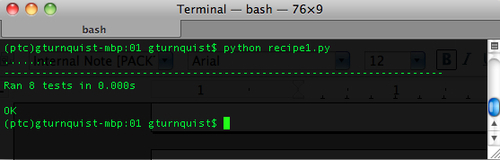4. Finally, verify that the environment is active by checking the path of `pip`.

### Tip

For more information on the usage and benefits of `virtualenv`, please read http://iamzed.com/2009/05/07/a-primer-on-virtualenv.

## Asserting the basics

The basic concept of an automated unittest test case is to instantiate part of our code, subject it to operations, and verify certain results using assertions.

• If the results are as expected, unittest counts it as a test success

• If the results don't match, an exception is thrown and unittest counts it as a test failure

Unittest was added to Python's standard batteries included library suite and doesn't require any extra installation.

### How to do it...

With these steps, we will code a simple program and then write some automated tests using unittest:

1. Create a new file called `recipe1.py` in which to put all of this recipe's code. Pick a class to test. This is known as the class under test. For this recipe, we'll pick a class that uses a simplistic Roman numeral converter:

```class RomanNumeralConverter(object):
def __init__(self, roman_numeral):
self.roman_numeral = roman_numeral
self.digit_map = {"M":1000, "D":500,  "C":100, "L":50, "X":10, "V":5, "I":1}

def convert_to_decimal(self):
val = 0
for char in self.roman_numeral:
val += self.digit_map[char]
return val```

### Note

This Roman numeral converter applies the simple rules of addition, but it doesn't have the special subtraction patterns such as `XL` mapping to `40`. The purpose is not to have the best Roman numeral converter, but to observe the various test assertions.

2. Write a new class and give it the same name with `Test` appended to the end, subclassing `unittest.TestCase`. Appending a test class with `Test` is a common convention, but not a requirement. Extending `unittest.TestCase` is a requirement needed to hook into unittest's standard test runner.

```import unittest

class RomanNumeralConverterTest(unittest.TestCase):```
3. Create several methods whose names start with `test`, so they are automatically picked up by the test number of unittest.

```  def test_parsing_millenia(self):
value = RomanNumeralConverter("M")
self.assertEquals(1000, value.convert_to_decimal())

def test_parsing_century(self):
value = RomanNumeralConverter("C")
self.assertEquals(100, value.convert_to_decimal())

def test_parsing_half_century(self):
value = RomanNumeralConverter("L")
self.assertEquals(50, value.convert_to_decimal())

value = RomanNumeralConverter("X")
self.assertEquals(10, value.convert_to_decimal())

value = RomanNumeralConverter("V")
self.assertEquals(5, value.convert_to_decimal())

def test_parsing_one(self):
value = RomanNumeralConverter("I")
self.assertEquals(1, value.convert_to_decimal())

def test_empty_roman_numeral(self):
value = RomanNumeralConverter("")
self.assertTrue(value.convert_to_decimal() == 0)
self.assertFalse(value.convert_to_decimal() > 0)

def test_no_roman_numeral(self):
value = RomanNumeralConverter(None)
self.assertRaises(TypeError, value.convert_to_decimal)```
4. Make the entire script runnable and then use unittest's test runner.

```if __name__ == "__main__":
unittest.main()```
5. Run the file from the command line.### How it works...

In the first step, we picked a class to test. Next, we created a separate test class. By naming the test class `[class under test]Test`, it is easy to tell which class is under test. Each test method name must start with `test`, so that unittest will automatically pick it up and run it. To add more tests, just define more `test` methods. Each of these tests utilizes various assertions.

• `assertEquals(first, second[, msg])`: Compares first and second expressions; and fails, if they don't have the same value. We can optionally print a special message if there is a failure.

• `assertTrue(expression[, msg])`: Tests the expression and fails if it is false. We can optionally print a special message if there is a failure.

• `assertFalse(expression[, msg])`: Tests the expression and fails if it is true. We can optionally print a special message if there is a failure.

• `assertRaises(exception, callable, …)`: Runs the callable, with any arguments, for the callable listed afterwards, and fails if it doesn't raise the exception.

### There's more...

Unittest provides many options for asserting, failing, and other convenient options. The following sections show some recommendations on how to pick and choose from these options.

#### assertEquals is preferred over assertTrue and assertFalse

When an `assertEquals` fails, the first and second values are printed in the error report, giving better feedback of what went wrong. `assertTrue` and `assertFalse` simply report failure. Not all testable results fit this but, if possible, use `assertEquals`.

It's important to understand the concept of equality. When comparing integers, strings, and other scalars, it's very simple. It doesn't work as well with collections like dictionaries, lists, and sets. Complex, custom-defined objects may carry custom definitions of equality. These complex objects may require more fine-grained assertions. That is why it's probably a good idea to also include some test methods that directly target equality and inequality when working with custom objects.

#### self.fail([msg]) can usually be rewritten with assertions

Unittest has a `self.fail([msg])` operation that unconditionally causes the test to fail, along with an optional message. This was not shown earlier because it is not recommended for use.

The `fail` method is often used to detect certain situations like exceptions. A common idiom is as follows:

```import unittest

def test_no_roman_numeral(self):
value = RomanNumeralConverter(None)
try:
value.convert_to_decimal()
self.fail("Expected a TypeError")
except TypeError, e:
pass```

This tests the same behavior as the earlier `test_no_roman_numeral`. The problem with this approach is that, when the code is working properly, the `fail` method is never executed. Code which is not executed regularly is at risk of becoming out of date and invalid. This will also interfere with coverage reports. Instead, it is better to use `assertRaises` as we used in the earlier examples. For other situations, look at rewriting the test using the other assertions.

#### Our version of Python can impact our options

Python's official documentation on unittest shows many other assertions, however, they depend on the version of Python we are using. Some have been deprecated; others are only available in later versions like Python 2.7.

If our code must support multiple versions of Python, then we must use the lowest common denominator. This recipe shows core assertions available in all versions since Python 2.1.

### Note

A newer unittest2 (http://pypi.python.org/pypi/unittest2/) is under development that backports several of these newer unittest features into Python 2.4+. However, due to unittest2 being in the beta stage at the time of writing and limitations to the size of this book, I decided to focus on unittest.

## Setting up and tearing down a test harness

Unittest provides an easy mechanism to configure the state of the system when a piece of code is put through a test. It also allows us to clean things up afterwards, if necessary. This is commonly needed when a particular test case has repetitive steps used in every test method.

Barring any references to external variables or resources that carry state from one test method to the next, each test method starts from the same state.

### How to do it...

With the following steps, we will setup and teardown a test harness for each test method.

1. Create a new file called `recipe2.py` in which to put all our code for this recipe.

2. Pick a class to test. In this case, we will use a slightly altered version of our Roman numeral converter, where the function, not the constructor, provides the input value to convert.

```class RomanNumeralConverter(object):
def __init__(self):
self.digit_map = {"M":1000, "D":500, "C":100, "L":50, "X":10, "V":5, "I":1}
def convert_to_decimal(self, roman_numeral):
val = 0
for char in roman_numeral:
val += self.digit_map[char]
return val```
3. Create a test class using the same name as the class under test with `Test` appended to the end.

```import unittest
class RomanNumeralConverterTest(unittest.TestCase):```
4. Create a `setUp` method that creates an instance of the class under test.

```  def setUp(self):
print "Creating a new RomanNumeralConverter..."
self.cvt = RomanNumeralConverter()```
5. Create a `tearDown` method that destroys the instance of the class under test.

```  def tearDown(self):
print "Destroying the RomanNumeralConverter..."
self.cvt = None```
6. Create all the test methods using `self.converter`.

```  def test_parsing_millenia(self):
self.assertEquals(1000, \
self.cvt.convert_to_decimal("M"))
def test_parsing_century(self):
self.assertEquals(100, \
self.cvt.convert_to_decimal("C"))
def test_parsing_half_century(self):
self.assertEquals(50, \
self.cvt.convert_to_decimal("L"))
self.assertEquals(10, \
self.cvt.convert_to_decimal("X"))
self.assertEquals(5, self.cvt.convert_to_decimal("V"))
def test_parsing_one(self):
self.assertEquals(1, self.cvt.convert_to_decimal("I"))
def test_empty_roman_numeral(self):
self.assertTrue(self.cvt.convert_to_decimal("") == 0)
self.assertFalse(self.cvt.convert_to_decimal("") > 0)
def test_no_roman_numeral(self):
self.assertRaises(TypeError, \
self.cvt.convert_to_decimal, None)```
7. Make the entire script runnable and then use the test runner of unittest.

```if __name__ == "__main__":
unittest.main()```
8. Run the file from the command line.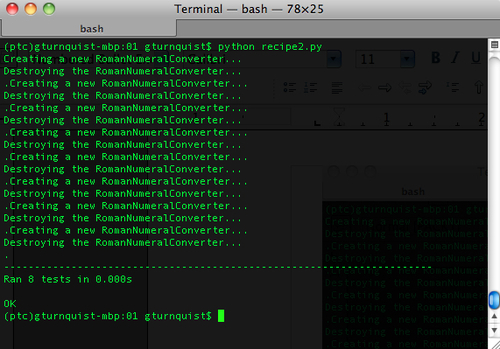### How it works...

In the first step, we picked a class to test. Next, we created a separate test class. By naming the test class `[class under test]Test`, it is easy to tell which class is under test.

Then, we defined a `setUp` method that unittest runs before every `test` method. Next, we created a `tearDown` method that unittest runs after every `test` method. In this case, we added a print statement in each of them to demonstrate unittest re-running these two methods for every test method. In reality, it would probably add too much noise to our testing.

One deficiency of unittest is the lack of `setUpClass/tearDownClass` and `setUpModule/tearDownModule`, providing the opportunity to run code in greater scopes than at the test method level. This has been added to unittest2, and has been described by some as handy, but won't be covered within the scope of this book.

### Tip

Each test case can have one setUp and one tearDown method

Our `RomanNumeralConverter` is pretty simple and fits easily into a single test class. But the test class allows only one `setUp` method and one `tearDown` method. If different combinations of `setUp`/`tearDown` methods are needed for various test scenarios, then this is a cue to code more test classes.

Just because we write a `setUp` method doesn't mean we need a `tearDown` method. In our case, we could have skipped destroying the `RomanNumeralConverter`, because a new instance would be replacing it for every test method. It was really for demonstration purposes only. What are the other uses of those cases which need a `tearDown` method? Using a library that requires some sort of `close` operation is a prime candidate for writing a `tearDown` method.

## Running test cases from the command line with increased verbosity

It is easy to adjust the test runner to print out every test method as it is run.

### How to do it...

In the following steps, we will run test cases with more detailed output, giving us better insight to how things run:

1. Create a new file called `recipe3.py` in which to store this recipe's code.

2. Pick a class to test. In this case, we will use our Roman numeral converter:

```class RomanNumeralConverter(object):
def __init__(self, roman_numeral):
self.roman_numeral = roman_numeral
self.digit_map = {"M":1000, "D":500, "C":100, "L":50, "X":10, "V":5, "I":1}

def convert_to_decimal(self):
val = 0
for char in self.roman_numeral:
val += self.digit_map[char]
return val```
3. Create a test class using the same name as the class under test with `Test` appended to the end.

```import unittest
class RomanNumeralConverterTest(unittest.TestCase):```
4. Create several test methods. For this recipe, the tests have been deliberately coded to fail.

```  def test_parsing_millenia(self):
value = RomanNumeralConverter("M")
self.assertEquals(1000, value.convert_to_decimal())

def test_parsing_century(self):
"This test method is coded to fail for demo."
value = RomanNumeralConverter("C")
self.assertEquals(10, value.convert_to_decimal())```
5. Define a test suite that automatically loads all the test methods, and then runs them with a higher level of verbosity.

```if __name__ == "__main__":
RomanNumeralConverterTest)
unittest.TextTestRunner(verbosity=2).run(suite)```
6. Run the file from the command line. Notice how the test method that fails prints out its Python docstring: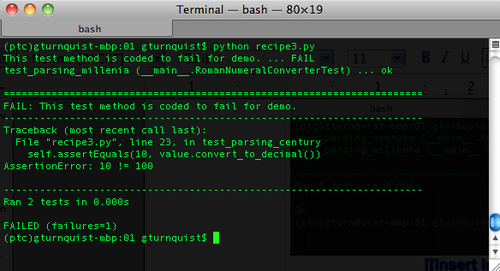### How it works...

A key part of automated testing is organizing the tests. The base units are called test cases. These can be combined together into test suites. Python's `unittest` module provides `TestLoader().loadTestsFromTestCase` to fetch all the `test*` methods automatically into a test suite. This test suite is then run through unittest's `TextTestRunner` with an increased level of verbosity.

### Note

`TextTestRunner` is unittest's only test runner. Later in this book, we will look at other test tools that have different runners, including one that plugs in a different unittest test runner.

The previous screenshot shows each method along with its module and class name, as well as success/failure.

### There's more...

This recipe not only demonstrates how to turn up the verbosity of running tests, but also shows what happens when a test case fails. It renames the `test` method with the document string embedded in the `test` method, and prints the details later after all the test methods have been reported.

## Running a subset of test case methods

Sometimes it's convenient to run only a subset of test methods in a given test case. This recipe will show how to run either the whole test case, or pick a subset from the command line.

### How to do it...

The following steps show how to code a command-line script to run subsets of tests:

1. Create a new file named `recipe4.py` in which to put all the code for this recipe.

2. Pick a class to test. In this case, we will use our `Roman` `numeral` `converter`.

```class RomanNumeralConverter(object):
def __init__(self, roman_numeral):
self.roman_numeral = roman_numeral
self.digit_map = {"M":1000, "D":500, "C":100, "L":50, "X":10, "V":5, "I":1}

def convert_to_decimal(self):
val = 0
for char in self.roman_numeral:
val += self.digit_map[char]
return val```
3. Create a test class using the same name as the class under test with `Test` appended to the end.

```import unittest
class RomanNumeralConverterTest(unittest.TestCase):```
4. Create several test methods.

```  def test_parsing_millenia(self):
value = RomanNumeralConverter("M")
self.assertEquals(1000, value.convert_to_decimal())

def test_parsing_century(self):
value = RomanNumeralConverter("C")
self.assertEquals(100, value.convert_to_decimal())```
5. Write a main runner that either runs the entire test case or accepts a variable number of test methods.

```if __name__ == "__main__":
import sys
suite = unittest.TestSuite()
if len(sys.argv) == 1:
RomanNumeralConverterTest)
else:
for test_name in sys.argv[1:]:
RomanNumeralConverterTest(test_name))

unittest.TextTestRunner(verbosity=2).run(suite)```
6. Run the recipe with no extra command-line arguments, and see it run all the tests. Also run it with a test method name, and see it run only the specified test method.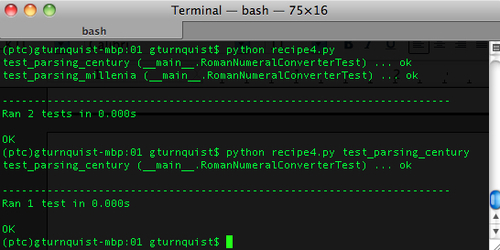### How it works...

For this test case, we coded a couple of test methods. But instead of simply running all the tests, or defining a fixed list, we used Python's `sys` library to parse the command-line arguments. If there are no extra arguments, it runs the entire test case. If there are extra arguments, then they are assumed to be test method names. It uses unittest's inbuilt ability to specify test method names when instantiating `RomanNumeralConverterTest`.

### Tip

Python 2.7 has this built in; Python 2.6 and earlier versions don't

Python 2.6 doesn't have this feature, which makes this recipe useful. If we are using Python 2.7, there is a command-line version we can use. If we need to support multiple versions of Python, this recipe can be quite handy.

## Chaining together a suite of tests

Unittest makes it easy to chain together test cases into a TestSuite. A TestSuite can be run just like a TestCase, but it also provides additional functionality to add a single test, multiple tests, and count them.

Why do we need this? Chaining together tests into a suite gives us the ability to pull together more than one module of test cases for a test run, as well as picking and choosing a subset of test cases. Up until now, we have generally run all the test methods from a single class. TestSuite gives us an alternative means to define a block of testing.

### How to do it...

In the following steps, we will code multiple test case classes, and then load their test methods into suites so we can run them.

1. Create a new file named `recipe5.py` in which to put our sample application and test cases.

2. Pick a class to test. In this case, we will use our Roman numeral converter.

```class RomanNumeralConverter(object):
def __init__(self):
self.digit_map = {"M":1000, "D":500, "C":100, "L":50, "X":10, "V":5, "I":1}

def convert_to_decimal(self, roman_numeral):
val = 0
for char in roman_numeral:
val += self.digit_map[char]
return val```
3. Create two test classes with various test methods spread between them.

```import unittest

class RomanNumeralConverterTest(unittest.TestCase):
def setUp(self):
self.cvt = RomanNumeralConverter()

def test_parsing_millenia(self):
self.assertEquals(1000, \
self.cvt.convert_to_decimal("M"))

def test_parsing_century(self):
self.assertEquals(100, \
self.cvt.convert_to_decimal("C"))

class RomanNumeralComboTest(unittest.TestCase):
def setUp(self):
self.cvt = RomanNumeralConverter()

def test_multi_millenia(self):
self.assertEquals(4000, \
self.cvt.convert_to_decimal("MMMM"))

self.assertEquals(2010, \
self.cvt.convert_to_decimal("MMX"))```
4. Create a test runner in a separate file named `recipe5_runner.py` that pulls in both test cases.

```if __name__ == "__main__":
import unittest
from recipe5 import *

RomanNumeralConverterTest)
RomanNumeralComboTest)
suite = unittest.TestSuite([suite1, suite2])
unittest.TextTestRunner(verbosity=2).run(suite)```
5. Execute the test runner, and observe how tests are pulled in from both test cases.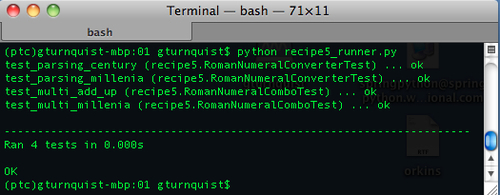### How it works...

The `unittest` module provides a convenient way to find all the test methods in a `TestClass` and bundle them together as a suite using its `loadTestsFromTestCase`. To further the usage of test suites, we are able to combine these two suites together as a single suite using `unittest.TestSuite([list...])`. The `TestSuite` class is designed to act like a `TestCase` class, even though it doesn't subclass `TestClass`, allowing us to run it using `TextTestRunner`. This recipe shows the verbosity turned up, allowing us to see exactly which test methods were run, and which test case they came from.

### There's more...

In this recipe, we ran the tests from a different file from where the test cases are defined. This is different from the previous recipes where the runnable code and the test case were contained in the same file. Since the runner is defining the tests we run, we can easily create more runners that combine different suites of tests.

#### Name of the test case should be significant

In the previous recipes, it has been advised to name the test case as `[class under test]Test`. This is to make it apparent to the reader that the class under test and the related test share an important relationship. Now that we are introducing another test case, we need to pick a different name. The name should explain clearly why these particular test methods are split out into a separate class. For this recipe, the methods are split out to show more complex combinations of Roman numerals.

## Defining test suites inside the test module

Each test module can provide one or more methods that define a different test suite. One method can exercise all the tests in a given module; another method can define a particular subset.

### How to do it...

With the following steps, we will create some methods that define test suites using different means:

1. Create a new file called `recipe6.py` in which to put our code for this recipe.

2. Pick a class to test. In this case, we will use our Roman numeral converter.

```class RomanNumeralConverter(object):
def __init__(self):
self.digit_map = {"M":1000, "D":500, "C":100, "L":50, "X":10, "V":5, "I":1}

def convert_to_decimal(self, roman_numeral):
val = 0
for char in roman_numeral:
val += self.digit_map[char]
return val```
3. Create a test class using the same name as the class under test with `Test` appended to the end.

```import unittest

class RomanNumeralConverterTest(unittest.TestCase):```
4. Write a series of test methods, including a `setUp` method that creates a new instance of the `RomanNumeralConverter` for each test method.

```import unittest

class RomanNumeralConverterTest(unittest.TestCase):
def setUp(self):
self.cvt = RomanNumeralConverter()

def test_parsing_millenia(self):
self.assertEquals(1000, \
self.cvt.convert_to_decimal("M"))

def test_parsing_century(self):
self.assertEquals(100, \
self.cvt.convert_to_decimal("C"))

def test_parsing_half_century(self):
self.assertEquals(50, \
self.cvt.convert_to_decimal("L"))

self.assertEquals(10, \
self.cvt.convert_to_decimal("X"))

self.assertEquals(5, self.cvt.convert_to_decimal("V"))

def test_parsing_one(self):
self.assertEquals(1, self.cvt.convert_to_decimal("I"))

def test_empty_roman_numeral(self):
self.assertTrue(self.cvt.convert_to_decimal("") == 0)
self.assertFalse(self.cvt.convert_to_decimal("") > 0)

def test_no_roman_numeral(self):
self.assertRaises(TypeError, \
self.cvt.convert_to_decimal, None)

def test_combo1(self):
self.assertEquals(4000, \
self.cvt.convert_to_decimal("MMMM"))

def test_combo2(self):
self.assertEquals(2010, \
self.cvt.convert_to_decimal("MMX"))

def test_combo3(self):
self.assertEquals(4668, \
self.cvt.convert_to_decimal("MMMMDCLXVIII"))```
5. Create some methods in the recipe's module (but not in the test case) that define different test suites.

```def high_and_low():
suite = unittest.TestSuite()
RomanNumeralConverterTest("test_parsing_millenia"))
RomanNumeralConverterTest("test_parsing_one"))
return suite

def combos():
return unittest.TestSuite(map(RomanNumeralConverterTest,\
["test_combo1", "test_combo2", "test_combo3"]))

def all():
RomanNumeralConverterTest)```
6. Create a runner that will iterate over each of these test suites and run them through unittest's `TextTestRunner`.

```if __name__ == "__main__":
for suite_func in [high_and_low, combos, all]:
print "Running test suite '%s'" % suite_func.func_name
suite = suite_func()
unittest.TextTestRunner(verbosity=2).run(suite)```
7. Run the combination of test suites, and see the results.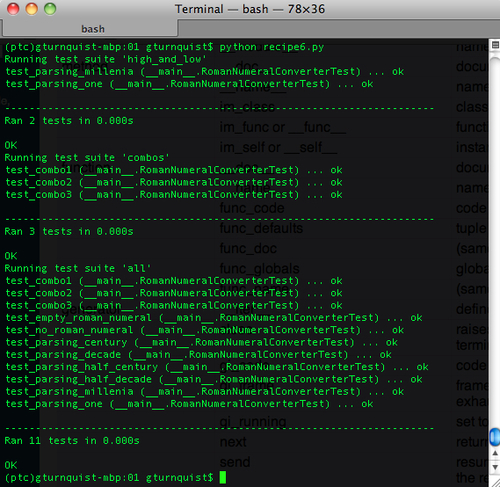### How it works...

We pick a class to test and define a number of test methods that check things out. Then we define a few module-level methods such as, `high_and_low`, `combos`, and `all`, to define test suites. Two of them contain fixed subsets of methods while `all` dynamically loads the `test*` methods from the class. Finally, the main part of our module iterates over a listing of all these functions that generate suites in order to smoothly create and run them.

### There's more...

All of our test suites were run from the recipe's main runner. But this probably wouldn't be the case for a real project. Instead, the idea is to define different suites, and code a mechanism to pick which suite to run. Each suite is geared towards a different purpose, and it is necessary to allow the developer to pick which suite to run. This can be done by coding a command-line script using Python's `optparse` module to define command-line flags to pick one of these suites.

#### Test suite methods must be outside of the test class

If we make these suite-defining methods members of the `test` class, we would have to instantiate the `test` class. Classes that extend `unittest.TestCase` have a specialized `init` method that doesn't work well with an instance that is created just to call a non-test method. That is why the methods are outside the `test` class. While these methods can be in other modules, it is very convenient to define them inside the module containing the test code, to keep things in proximity.

#### Why have different suites?

What if we started our project off by running all tests? Sounds like a good idea, right? But what if the time to run the entire test suite grew to over an hour? There is a certain threshold after which developers tend to stop running tests, and nothing is worse than an un-run test suite. By defining subsets of tests, it is easy to run alternate suites during the day, and then perhaps run the comprehensive test suite once a day.

• `all` is the comprehensive suite

• `high_and_low` is an example of testing the edges

• `combos` is a random sampling of values used to show that things are generally working

Defining our test suites is a judgment call. It's also worth it to re-evaluate each test suite every so often. If one test suite is getting too costly to run, consider moving some of its more expensive tests to another suite.

#### optparse is being phased out and replaced by argparse

While `optparse` is a convenient way to add command-line flags to Python scripts, it won't be available forever. Python 2.7 has deprecated this module and is continuing this development in `argparse`.

## Retooling old test code to run inside unittest

Sometimes, we may have developed demo code to exercise our system. We don't have to rewrite it to run it inside unittest. Instead, it is easy to hook it up to the test framework and run it with some small changes.

### How to do it...

With these steps, we will dive into capturing the test code that was written without using unittest, and repurposing it with minimal effort to run inside unittest.

1. Create a file named `recipe7.py` in which to put our application code that we will be testing.

2. Pick a class to test. In this case, we will use our Roman numeral converter.

```class RomanNumeralConverter(object):
def __init__(self):
self.digit_map = {"M":1000, "D":500, "C":100, "L":50, "X":10, "V":5, "I":1}

def convert_to_decimal(self, roman_numeral):
val = 0
for char in roman_numeral:
val += self.digit_map[char]
return val```
3. Create a new file named `recipe7_legacy.py` to contain test code that doesn't use the `unittest` module.

4. Create a set of legacy tests that are coded, based on Python's `assert` function, not with unittest, along with a runner.

```from recipe7 import *

class RomanNumeralTester(object):
def __init__(self):
self.cvt = RomanNumeralConverter()

def simple_test(self):
print "+++ Converting M to 1000"
assert self.cvt.convert_to_decimal("M") == 1000

def combo_test1(self):
print "+++ Converting MMX to 2010"
assert self.cvt.convert_to_decimal("MMXX") == 2010

def combo_test2(self):
print "+++ Converting MMMMDCLXVIII to 4668"
val = self.cvt.convert_to_decimal("MMMMDCLXVII")
self.check(val, 4668)

def other_test(self):
print "+++ Converting MMMM to 4000"
val = self.cvt.convert_to_decimal("MMMM")
self.check(val, 4000)

def check(self, actual, expected):
if (actual != expected):
raise AssertionError("%s doesn't equal %s" % \
(actual, expected))

def test_the_system(self):
self.simple_test()
self.combo_test1()
self.combo_test2()
self.other_test()

if __name__ == "__main__":
tester = RomanNumeralTester()
tester.test_the_system()```

### Note

This set of legacy tests is meant to represent legacy test code that our team has developed to exercise things before unittest was an option.

5. Run the legacy tests. What is wrong with this situation? Did all the test methods run? Have we caught all the bugs?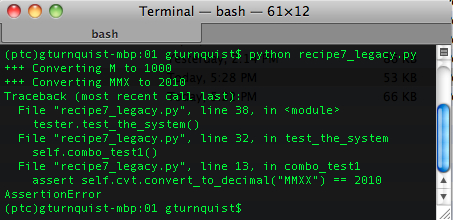6. Create a new file called `recipe7_pyunit.py`.

7. Create a unittest set of tests, wrapping each legacy test method inside unittest's `FunctionTestCase`.

```from recipe7 import *
from recipe7_legacy import *
import unittest

if __name__ == "__main__":
tester = RomanNumeralTester()

suite = unittest.TestSuite()
for test in [tester.simple_test, tester.combo_test1, \
tester.combo_test2, tester.other_test]:
testcase = unittest.FunctionTestCase(test)

unittest.TextTestRunner(verbosity=2).run(suite)```
8. Run the unittest test. Did all the tests run this time? Which test failed? Where is the bug?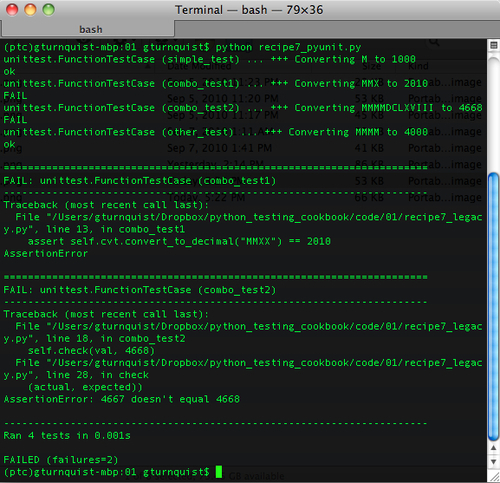### How it works...

Python provides a convenient `assert` statement that tests a condition. When true, the code continues. When false, it raises an `AssertionError`. In the first test runner, we have several tests that check results using a mixture of `assert` statements or raising an `AssertionError`.

unittest provides a convenient class, `unittest.FunctionTestCase`, that wraps a bound function as a unittest test case. If an `AssertionError` is thrown, `FunctionTestCase` catches it, flags it as a test failure, and proceeds to the next test case. If any other type of exception is thrown, it will be flagged as a test error. In the second test runner, we wrap each of these legacy test methods with `FunctionTestCase`, and chain them together in a suite for unittest to run.

As seen by running the second test run, there is a bug lurking in the third test method. We were not aware of it, because the test suite was prematurely interrupted.

Another deficiency of Python's `assert` statement is shown by the first failure, as seen in the previous screenshot. When an assert fails, there is little to no information about the values that were compared. All we have is the line of code where it failed. The second assert in that screenshot was more useful, because we coded a custom checker that threw a custom `AssertionError`.

### There's more...

Unittest does more than just run tests. It has a built-in mechanism to trap errors and failures, and then it continues running as much of our test suite as possible. This helps, because we can shake out more errors and fix more things within a given test run. This is especially important when a test suite grows to the point of taking minutes or hours to run.

#### Where are the bugs?

They exist in the test methods, and fundamentally were made by making slight alterations in the Roman numeral being converted.

```  def combo_test1(self):
print "+++ Converting MMX to 2010"
assert self.cvt.convert_to_decimal("MMXX") == 2010

def combo_test2(self):
print "+++ Converting MMMMDCLXVIII to 4668"
val = self.cvt.convert_to_decimal("MMMMDCLXVII")
self.check(val, 4668)```

The `combo_test1` test method prints out that it is converting `MMX`, but actually tries to convert `MMXX`. The `combo_test2` test method prints out that it is converting `MMMMDCLXVIII`, but actually tries to convert `MMMMDCLXVII`.

This is a contrived example, but have you ever run into bugs just as small that drove you mad trying to track them down? The point is, showing how easy or hard it can be to track them down is based on how the values are checked. Python's `assert` statement isn't very effective at telling us what values are compared where. The customized `check` method is much better at pinpointing the problem with `combo_test2`.

### Tip

This highlights the problem with having comments or print statements trying to reflect what the asserts do. They can easily get out of sync and the developer may face some problems trying to track down bugs. Avoiding this situation is known as the DRY principle (Don't Repeat Yourself).

#### FunctionTestCase is a temporary measure

The `FunctionTestCase` is a test case that provides an easy way to quickly migrate tests based on Python's `assert` statement, so they can be run with unittest. But things shouldn't stop there. If we take the time to convert `RomanNumeralTester` into a unittest `TestCase`, then we gain access to other useful features like the various `assert*` methods that come with `TestCase`. It's a good investment. The `FunctionTestCase` just lowers the bar to migrate to unittest.

## Breaking down obscure tests into simple ones

Unittest provides the means to test the code through a series of assertions. I have often felt the temptation to exercise many aspects of a particular piece of code within a single test method. If any part fails, it becomes obscured as to which part failed. It is preferable to split things up into several smaller test methods, so that when some part of the code under test fails, it is obvious.

### How to do it...

With these steps, we will investigate what happens when we put too much into a single test method.

1. Create a new file named `recipe8.py` in which to put our application code for this recipe.

2. Pick a class to test. In this case, we will use an alternative version of the Roman numeral converter, which converts both ways.

```class RomanNumeralConverter(object):
def __init__(self):
self.digit_map = {"M":1000, "D":500, "C":100, "L":50, "X":10, "V":5, "I":1}

def convert_to_decimal(self, roman_numeral):
val = 0
for char in roman_numeral:
val += self.digit_map[char]
return val

def convert_to_roman(self, decimal):
val = ""
while decimal > 1000:
val += "M"
decimal -= 1000
while decimal > 500:
val += "D"
decimal -= 500
while decimal > 100:
val += "C"
decimal -= 100
while decimal > 50:
val += "L"
decimal -= 50
while decimal > 10:
val += "X"
decimal -= 10
while decimal > 5:
val += "V"
decimal -= 5
while decimal > 1:
val += "I"
decimal -= 1
return val```
3. Create a new file called `recipe8_obscure.py` in which to put some longer test methods.

4. Create some test methods that combine several test assertions.

```import unittest
from recipe8 import *

class RomanNumeralTest(unittest.TestCase):
def setUp(self):
self.cvt = RomanNumeralConverter()

def test_convert_to_decimal(self):
self.assertEquals(0, self.cvt.convert_to_decimal(""))
self.assertEquals(1, self.cvt.convert_to_decimal("I"))
self.assertEquals(2010, \
self.cvt.convert_to_decimal("MMX"))
self.assertEquals(4000, \
self.cvt.convert_to_decimal("MMMM"))

def test_convert_to_roman(self):
self.assertEquals("", self.cvt.convert_to_roman(0))
self.assertEquals("II", self.cvt.convert_to_roman(2))
self.assertEquals("V", self.cvt.convert_to_roman(5))
self.assertEquals("XII", \
self.cvt.convert_to_roman(12))
self.assertEquals("MMX", \
self.cvt.convert_to_roman(2010))
self.assertEquals("MMMM", \
self.cvt.convert_to_roman(4000))

if __name__ == "__main__":
unittest.main()```
5. Run the obscure tests. Why did it fail? Where is the bug? It reports that `II` is not equal to `I`, so something appears to be off. If this the only bug?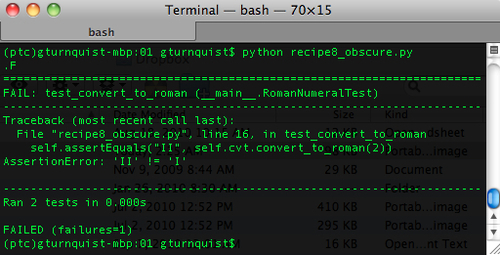6. Create another file called `recipe8_clear.py` to create a more fine-grained set of test methods.

7. Split up the assertions into separate test methods to give a higher fidelity of output.

```import unittest
from recipe8 import *

class RomanNumeralTest(unittest.TestCase):
def setUp(self):
self.cvt = RomanNumeralConverter()

def test_to_decimal1(self):
self.assertEquals(0, self.cvt.convert_to_decimal(""))

def test_to_decimal2(self):
self.assertEquals(1, self.cvt.convert_to_decimal("I"))

def test_to_decimal3(self):
self.assertEquals(2010, \
self.cvt.convert_to_decimal("MMX"))

def test_to_decimal4(self):
self.assertEquals(4000, \
self.cvt.convert_to_decimal("MMMM"))

def test_convert_to_roman1(self):
self.assertEquals("", self.cvt.convert_to_roman(0))

def test_convert_to_roman2(self):
self.assertEquals("II", self.cvt.convert_to_roman(2))

def test_convert_to_roman3(self):
self.assertEquals("V", self.cvt.convert_to_roman(5))

def test_convert_to_roman4(self):
self.assertEquals("XII", \
self.cvt.convert_to_roman(12))

def test_convert_to_roman5(self):
self.assertEquals("MMX", \
self.cvt.convert_to_roman(2010))

def test_convert_to_roman6(self):
self.assertEquals("MMMM", \
self.cvt.convert_to_roman(4000))

if __name__ == "__main__":
unittest.main()```
8. Run the clearer test suite. Is it a bit clearer where the bug is? What did we trade in to get this higher degree of test failure? Was it worth the effort?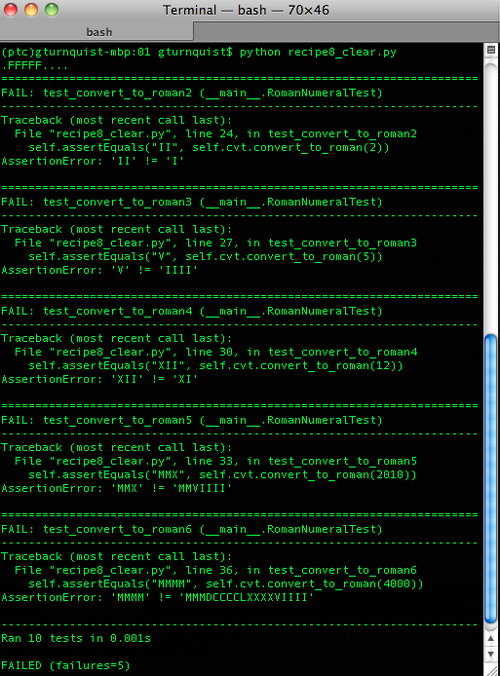### How it works...

In this case, we created a modified Roman numeral converter that converts both ways. We then started creating test methods to exercise things. Since each of these tests were a simple, one-line assertion, it was convenient to put them all in the same test method.

In the second test case, we put each assertion into a separate test method. Running it exposes the fact that there are multiple bugs in this Roman numeral converter.

### There's more...

When we started off writing tests, it was very convenient to bundle all these assertions into a single test method. After all, if everything is working, there is no harm, right? But what if everything does not work, what do we have to deal with? An obscure error report!

#### Where is the bug?

The obscured test runner may not be clear. All we have to go on is `II` `!=` `I`. Not much. The clue is that it is only off by one. The clear test runner gives more clues. We see that `V !=` `IIII`, `XII` `!=` `XI`, and some more. Each of these failures shows things being off by one.

The bug involves the various Boolean conditions in the while checks:

```    while decimal > 1000:
while decimal > 500:
while decimal > 100:
while decimal > 50:
while decimal > 10:
while decimal > 5:
while decimal > 1:```

Instead of testing greater than, it should test for greater than or equal to. This causes it to skip out of each Roman numeral before counting the last one.

#### What is the right size for a test method?

In this recipe, we broke things down to a single assertion per test. But I wouldn't advise thinking along these lines.

If we look a little closer, each test method also involves a single usage of the Roman numeral API. For the converter, there is only one result to examine when exercising the code. For other systems, the output may be more complex. It is completely warranted to use several assertions in the same test method to check the outcome by making that single call.

When we proceed to make more calls to the Roman numeral API, it should signal us to consider splitting it off into a new test method.

This opens up the question: what is a unit of code? There has been much debate over what defines a unit of code, and what makes a good unit test. There are many opinions. Hopefully, reading this chapter and weighing it against the other test tactics covered throughout this book will help you enhance your own opinion and ultimately improve your own testing talent.

#### Unittests versus integration tests

Unittest can easily help us write both unit tests as well as integration tests. Unit tests exercise smaller blocks of code. When writing unit tests, it is best to keep the testing as small and fine grained as possible.

When we move up to a higher level (such as integration testing), it makes sense to test multiple steps in a single test method. But this is only recommended if there are adequate low-level unit tests. This will shed some light on whether it is broken at the unit level, or whether there is a sequence of steps that causes the error.

Integration tests often extend to things like external systems. For example, many argue that unit testing should never connect to a database, talk to an LDAP server, or interact with other systems.

### Tip

Just because we are using unittest, it doesn't mean the tests we are writing are unit tests. Later in this book, we will visit the concept that unittest can be used to write many types of tests including integration tests, smoke tests, and other types of tests as well.

## Testing the edges

When we write automated tests, we pick the inputs and assert the expected outputs. It is important to test the limits of the inputs to make sure our code can handle good and bad inputs. This is also known as testing corner cases.

### How to do it...

As we dig into this recipe, we will look for good boundaries to test against.

1. Create a new file named `recipe9.py` in which to put all our code for this recipe.

2. Pick a class to test. In this recipe, we'll use another variation of our Roman numeral converter. This one doesn't process values greater than 4000.

```class RomanNumeralConverter(object):
def __init__(self):
self.digit_map = {"M":1000, "D":500, "C":100, "L":50, "X":10, "V":5, "I":1}

def convert_to_decimal(self, roman_numeral):
val = 0
for char in roman_numeral:
val += self.digit_map[char]
if val > 4000:
raise Exception("We don't handle values over 4000")
return val

def convert_to_roman(self, decimal):
if decimal > 4000:
raise Exception("We don't handle values over 4000")
val = ""
mappers = [(1000,"M"), (500,"D"), (100,"C"), (50,"L"), (10,"X"), (5,"V"), (1,"I")]
for (mapper_dec, mapper_rom) in mappers:
while decimal >= mapper_dec:
val += mapper_rom
decimal -= mapper_dec
return val```
3. Create a test case that sets up an instance of the Roman numeral converter.

```import unittest

class RomanNumeralTest(unittest.TestCase):
def setUp(self):
self.cvt = RomanNumeralConverter()```
4. Add several test methods that exercise the edges of converting to Roman numeral notation.

```  def test_to_roman_bottom(self):
self.assertEquals("I", self.cvt.convert_to_roman(1))

def test_to_roman_below_bottom(self):
self.assertEquals("", self.cvt.convert_to_roman(0))

def test_to_roman_negative_value(self):
self.assertEquals("", self.cvt.convert_to_roman(-1))

def test_to_roman_top(self):
self.assertEquals("MMMM", \
self.cvt.convert_to_roman(4000))

def test_to_roman_above_top(self):
self.assertRaises(Exception, \
self.cvt.convert_to_roman, 4001)```
5. Add several test methods that exercise the edges of converting to decimal notation.

```  def test_to_decimal_bottom(self):
self.assertEquals(1, self.cvt.convert_to_decimal("I"))

def test_to_decimal_below_bottom(self):
self.assertEquals(0, self.cvt.convert_to_decimal(""))

def test_to_decimal_top(self):
self.assertEquals(4000, \
self.cvt.convert_to_decimal("MMMM"))

def test_to_decimal_above_top(self):
self.assertRaises(Exception, \
self.cvt.convert_to_decimal, "MMMMI")```
6. Add some tests that exercise the tiers of converting decimals to Roman numerals.

```  def test_to_roman_tier1(self):
self.assertEquals("V", self.cvt.convert_to_roman(5))

def test_to_roman_tier2(self):
self.assertEquals("X", self.cvt.convert_to_roman(10))

def test_to_roman_tier3(self):
self.assertEquals("L", self.cvt.convert_to_roman(50))

def test_to_roman_tier4(self):
self.assertEquals("C", self.cvt.convert_to_roman(100))

def test_to_roman_tier5(self):
self.assertEquals("D", self.cvt.convert_to_roman(500))

def test_to_roman_tier6(self):
self.assertEquals("M", \
self.cvt.convert_to_roman(1000))```
7. Add some tests that input unexpected values to the Roman numeral converter.

```  def test_to_roman_bad_inputs(self):
self.assertEquals("", self.cvt.convert_to_roman(None))
self.assertEquals("I", self.cvt.convert_to_roman(1.2))

self.assertRaises(TypeError, \
self.cvt.convert_to_decimal, None)
self.assertRaises(TypeError, \
self.cvt.convert_to_decimal, 1.2)```
8. Add a unit test runner.

```if __name__ == "__main__":
unittest.main()```
9. Run the test case.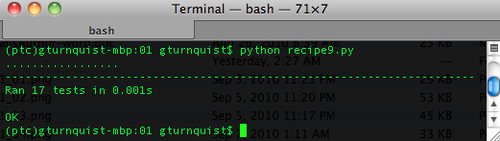### How it works...

We have a specialized Roman numeral converter that only converts values up to `MMMM` or `4000`. We have written several test methods to exercise it. The immediate edges we write tests for are `1` and `4000`. We also write some tests for one step past that: `0` and `4001`. To make things complete, we also test against `-1`.

### There's more...

A key part of the algorithm involves handling the various tiers of Roman numerals (5, 10, 50, 100, 500, and 1000). These could be considered mini-edges, so we wrote tests to check that the code handled those as well. Do you think we should test one past the mini-edges?

It's recommended that we should. Many bugs erupt due to coding greater than, when it should be greater than or equal (or vice versa), and so on. Testing one past the boundary, in both directions, is the perfect way to make sure that things are working exactly as expected. We also need to check bad inputs, so we tried converting `None` and a `float`.

That previous statement raises an important question: how many invalid types should we test against? Because Python is dynamic, we can expect a lot of input types. So what is reasonable? If our code hinges on a dictionary lookup, like certain parts of our Roman numeral API does, then confirming that we correctly handle a KeyError would probably be adequate. We don't need to input lots of different types if they all result in a KeyError.

#### Identifying the edges is important

It's important to identify the edges of our system, because we need to know our software can handle these boundaries. We also need to know it can handle both sides of these boundaries that are good values and bad values. That is why we need to check `4000` and `4001`, as well as `0` and `1`. This is a common place where software breaks.

#### Testing for unexpected conditions

Does this sound a little awkward? Expect the unexpected? Our code involves converting integers and strings back and forth. By 'unexpected', we mean types of inputs passed in when someone uses our library that doesn't understand the edges, or wires it to receive inputs that are wider ranging types than we expected to receive.

A common occurrence of misuse is when a user of our API is working against a collection such as a list and accidentally passes the entire list instead of a single value by iteration. Another often seen situation is when a user of our API passes in `None` due to some other bug in their code. It's good to know that our API is resilient enough to handle this.

## Testing corner cases by iteration

While developing code, new corner case inputs are often discovered. Being able to capture these inputs in an iterable array makes it easy to add related test methods.

### How to do it...

In this recipe, we will look at a different way to test corner cases.

1. Create a new file called `recipe10.py` in which to put all our code for this recipe.

2. Pick a class to test. In this recipe, we'll use another variation of our Roman numeral converter. This one doesn't process values greater than 4000.

```class RomanNumeralConverter(object):
def __init__(self):
self.digit_map = {"M":1000, "D":500, "C":100, "L":50, "X":10, "V":5, "I":1}

def convert_to_decimal(self, roman_numeral):
val = 0
for char in roman_numeral:
val += self.digit_map[char]
if val > 4000:
raise Exception(\
"We don't handle values over 4000")
return val

def convert_to_roman(self, decimal):
if decimal > 4000:
raise Exception(\
"We don't handle values over 4000")
val = ""
mappers = [(1000,"M"), (500,"D"), (100,"C"), (50,"L"), (10,"X"), (5,"V"), (1,"I")]
for (mapper_dec, mapper_rom) in mappers:
while decimal >= mapper_dec:
val += mapper_rom
decimal -= mapper_dec
return val```
3. Create a test class to exercise the Roman numeral converter.

```import unittest

class RomanNumeralTest(unittest.TestCase):
def setUp(self):
self.cvt = RomanNumeralConverter()```
4. Write a test method that exercises the edges of the Roman numeral converter.

```  def test_edges(self):
r = self.cvt.convert_to_roman
d = self.cvt.convert_to_decimal
edges = [("equals", r, "I", 1),\
("equals", r, "", 0),\
("equals", r, "", -1),\
("equals", r, "MMMM", 4000),\
("raises", r, Exception, 4001),\
("equals", d, 1, "I"),\
("equals", d, 0, ""),\
("equals", d, 4000, "MMMM"),\
("raises", d, Exception, "MMMMI")
]

[self.checkout_edge(edge) for edge in edges]```
5. Create a test method that exercises the tiers converting from decimal to Roman numerals.

```  def test_tiers(self):
r = self.cvt.convert_to_roman
edges = [("equals", r, "V", 5),\
("equals", r, "VIIII", 9),\
("equals", r, "X", 10),\
("equals", r, "XI", 11),\
("equals", r, "XXXXVIIII", 49),\
("equals", r, "L", 50),\
("equals", r, "LI", 51),\
("equals", r, "LXXXXVIIII", 99),\
("equals", r, "C", 100),\
("equals", r, "CI", 101),\
("equals", r, "CCCCLXXXXVIIII", 499),\
("equals", r, "D", 500),\
("equals", r, "DI", 501),\
("equals", r, "M", 1000)\
]

[self.checkout_edge(edge) for edge in edges]```
6. Create a test method that exercises a set of invalid inputs.

```  def test_bad_inputs(self):
r = self.cvt.convert_to_roman
d = self.cvt.convert_to_decimal
edges = [("equals", r, "", None),\
("equals", r, "I", 1.2),\
("raises", d, TypeError, None),\
("raises", d, TypeError, 1.2)\
]

[self.checkout_edge(edge) for edge in edges]```
7. Code a utility method that iterates over the edge cases and runs different assertions based on each edge.

```  def checkout_edge(self, edge):
if edge == "equals":
f, output, input = edge, edge, edge
print("Converting %s to %s..." % (input, output))
self.assertEquals(output, f(input))
elif edge == "raises":
f, exception, args = edge, edge, edge[3:]
print("Converting %s, expecting %s" % \
(args, exception))
self.assertRaises(exception, f, *args)```
8. Make the script runnable by loading the test case into `TextTestRunner`.

```if __name__ == "__main__":
RomanNumeralTest)
unittest.TextTestRunner(verbosity=2).run(suite)```
9. Run the test case.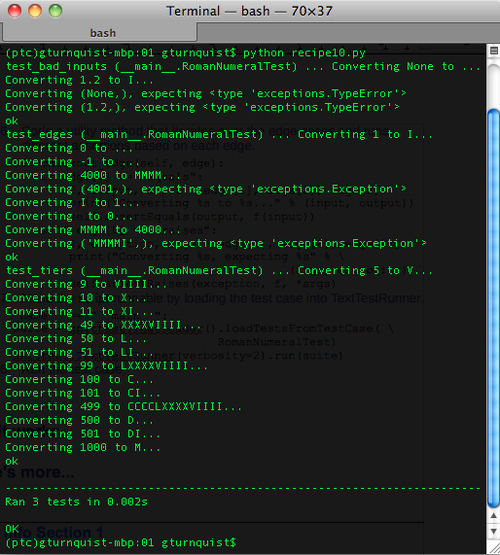### How it works...

We have a specialized Roman numeral converter that only converts values up to `MMMM` or `4000`. The immediate edges which we write tests for are `1` and `4000`. We also write some tests for one step past that: `0` and `4001`. To make things complete, we also test against `-1`.

But we've written the tests a little differently. Instead of writing each test input/output combination as a separate test method, we capture the input and output values in a tuple that is embedded in a list. We then feed it to our test iterator, `checkout_edge`. Because we need both `assertEquals` and `assertRaises` calls, the tuple also includes either `equals` or `raises` to flag which assertion to use.

Finally, to make it flexibly handle the convertion of both Roman numerals and decimals, the handles on the `convert_to_roman` and `convert_to_decimal` functions of our Roman numeral API is embedded in each tuple as well.

As shown in the following highlighted parts, we grab a handle on `convert_to_roman`, and store it in `r`. Then we embed it in the third element of the highlighted tuple, allowing the `checkout_edge` function to call it when needed.

```  def test_bad_inputs(self):
r = self.cvt.convert_to_roman
d = self.cvt.convert_to_decimal
edges = [("equals", r, "", None),\
("equals", r, "I", 1.2),\
("raises", d, TypeError, None),\
("raises", d, TypeError, 1.2)\
]

[self.checkout_edge(edge) for edge in edges]```

### There's more...

A key part of the algorithm involves handling the various tiers of Roman numerals (5, 10, 50, 100, 500, and 1000). These could be considered mini-edges, so we wrote a separate test method that has a list of input/output values to check those out as well. In the recipe Testing the edges, we didn't include testing before and after these mini-edges, for example `4` and `6` for `5`. Now that it only takes one line of data to capture this test, we have it in this recipe. The same was done for all the others (except 1000).

Finally, we need to check bad inputs, so we created one more test method where we try to convert `None` and a `float` to and from Roman numeral.

#### Does this defy the recipe—Breaking down obscure tests into simple ones?

In a way, it does. If something goes wrong in one of the test data entries, then that entire test method will have failed. That is one reason why the other recipe split things up into three test methods instead of one big test method to cover them all. This is a judgment call about when it makes sense to view inputs and outputs as more data than test method. If you find the same sequence of test steps occurring repeatedly, consider whether it makes sense to capture the values in some sort of table structure, like the list used in this recipe.

#### How does this compare with the recipe—Testing the edges?

In case it wasn't obvious, these are the exact same tests used in the recipe Testing the edges. The question is, which version do you find more readable? Both are perfectly acceptable. Breaking things up into separate methods makes it more fine-grained and easier to spot if something goes wrong. Collecting things together into a data structure, the way we did in this recipe makes it more succinct, and could spur us on to write more test combinations as we did for the conversion tiers.

In my opinion, when testing algorithmic functions that have simple inputs and outputs, it's more suitable to use this recipe's mechanism to code an entire battery of test inputs in this concise format, for example, a mathematical function, a sorting algorithm, or perhaps a transform function.

When testing functions that are more logical and imperative, the other recipe may be more useful. For example, functions that interact with a database, cause changes in the state of the system, or other types of side effects that aren't encapsulated in the return value, would be hard to capture using this recipe.